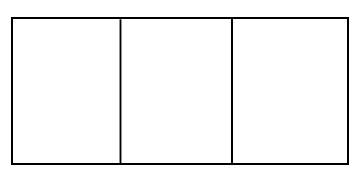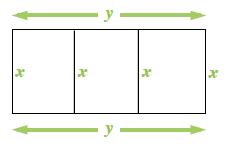### Home > PC > Chapter 7 > Lesson 7.3.2 > Problem7-112

7-112.A rectangular pen is to be constructed with three sections as shown in the diagram. If the total amount of fencing available is $500$ feet, what is the maximum area that can be enclosed by the fence? (It is not necessary for the three sections to have equal areas.)

Label the diagram.

Write an equation for the perimeter and the available $500$ feet of fencing. Solve for $y$.Write an equation for the area in terms of $x$ and $y$.

Substitute the expression for $y$ from the perimeter equation into the area equation.

Use your calculator to graph the area function.